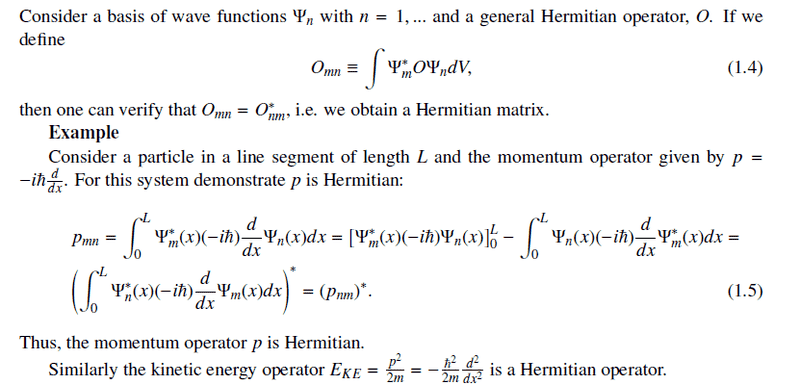# Verify that this kinetic energy operator is Hermitian

## Homework Statement

Not actually a homework question but is an exercise in my lecture notes.

## Homework Equations

I'm following this which demonstrates that the momentum operator is Hermitian:## The Attempt at a Solution

$$KE_{mn} = (\frac{-\hbar^2}{2m}) \int\Psi_{m}^{*} \Psi_{n}^{''} dx$$
$$by parts: \int uv' = uv - \int vu'$$
$$KE_{mn} = (\frac{-\hbar^2}{2m}) \Big( \Psi_{m}^* \Psi_{n}^{'} - \int \Psi_{n}^{'} \Psi_{m}^{'*} dx \Big)$$
$$KE_{mn} = (\frac{-\hbar^2}{2m}) \Big( \Psi_{m}^* \Psi_{n}^{'} - (\Psi_{n}^{'} \Psi_{m}^{*} - \int \Psi_{m}^{*}\Psi_{n}^{''} dx) \Big)$$
$$KE_{mn} = (\frac{-\hbar^2}{2m}) \int \Psi_{m}^{*}\Psi_{n}^{''} dx$$
$$KE_{mn} = KE_{mn}$$Can anyone see the gaping error in my working?

Thanks## Answers and Replies

DrClaude
Mentor
$$KE_{mn} = (\frac{-\hbar^2}{2m}) \Big( \Psi_{m}^* \Psi_{n}^{'} - \int \Psi_{n}^{'} \Psi_{m}^{'*} dx \Big)$$
$$KE_{mn} = (\frac{-\hbar^2}{2m}) \Big( \Psi_{m}^* \Psi_{n}^{'} - (\Psi_{n}^{'} \Psi_{m}^{*} - \int \Psi_{m}^{*}\Psi_{n}^{''} dx) \Big)$$
The second time you are doing the integration by parts, you are making the wrong choice for ##u## and ##v'##.

Also, don't forget that this is a definite integral.

•sa1988
The second time you are doing the integration by parts, you are making the wrong choice for ##u## and ##v'##.

Also, don't forget that this is a definite integral.

Thanks for this. Relieving to see it was a fairly simple arithmetic error, but worrying that my eyes continually didn't pick up on it...

Pretty late in the evening now and I don't have any pen or paper handy for going over it all, but I'll check it out tomorrow.

Cheers :)

Hi, may I have the full set solution of this question? Thank you

PeroK
Science Advisor
Homework Helper
Gold Member
2020 Award
Hi, may I have the full set solution of this question? Thank you

Okay, but maybe you are two years too late!

Personally, I would have used the Hermitian property of ##p## to show the Hermitian property of ##p^2## directly.

DrClaude
Mentor
Hi, may I have the full set solution of this question? Thank you
We don't give full solutions at PhysicsForums. Try it yourself and create a thread with your question if you are having some problems. We will help you get to the solution.